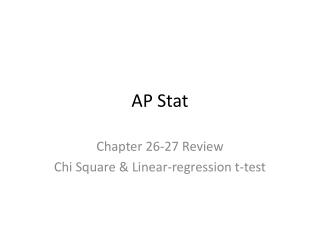# AP Stat - PowerPoint PPT PresentationDownload PresentationAP Stat

AP StatDownload Presentation## AP Stat

- - - - - - - - - - - - - - - - - - - - - - - - - - - E N D - - - - - - - - - - - - - - - - - - - - - - - - - - -
##### Presentation Transcript

1. AP Stat Chapter 26-27 Review Chi Square & Linear-regression t-test

2. 1. You wonder if a bag of M & M’s is made up of 1/6 orange, brown, yellow, green, blue & red. • Your friend weights each color in the bag and finds 10 grams of orange, 11 grams of brown, • 9 grams of yellow, 7 grams of green, 13 grams of blue & 10 grams of red. • What could you conclude from running a Chi Squared test of the expected colors • of M & M’s? • Answer: Can’t do a Chi Squared because M & M’s were not counted. • How many df are there for a chi-square test of homogeneity based on a table that has • 5 rows & 6 columns? • Answer:df = 20 • Find the number of times you expect each face of a dice to come up if you roll it 90 times. • Answer: 15

3. A new blood pressure medication is tested on 30 volunteers from 5 different age groups. The • Results are shown on the table above. • 4. If a Chi-square test were done, what kind of test would be conducted? • Answer: Chi-square test of homogeneity • 5. Find the expected number of successful cases of 40-49 year olds. • Answer: (30/150) x 70 = 14 • 6. Suppose we are testing an SAT prep program. At the end we want to see if the amount • of points gained depends on the number of hours a student spent preparing for the SAT. • What kind of test would we do? • Answer: Linear Regression t-test

4. Trix cereal comes in five fruit flavors, and each flavor has a different shape. A curious Student methodically sorted an entire box of the cereal & found the distribution of flavors For the pieces of cereal in the box as shown above: Is there evidence that the flavors are evenly distributed? P: The distribution of the different flavors in a box of Trix H: Ho: The flavors are evenly distributed Ha: The flavors in the box are not evenly distributed. A: Expected Counts: 2615/5 = 523 for each flavor ECF’s >5, data is counted data, sample is representative of a typical box of Trix N: Chi-square GOF test T: ECF’s shown above, df = 4

5. O: M: Reject Ho at the .05 level S: There is strong evidence that the distribution of Trix is not the same by flavor. Orange & strawberry have more than expected & lemon & lime have less than expected.

6. A sample of the non-academic interests of students at a high school is shown below. The observed counts are shown versus the expected counts • Show how this distribution would be changed to meet the ECF condition

7. A hypothesis test is run where the null hypothesis is the distribution of non-academic activities is the same across grade levels against the alternative hypothesis that the distribution of non-academic activities is not the same across grade levels. • What kind of test is this? • Answer: χ2 Homogeneity Test • The results of the test are shown below. State the conclusion. • Answer: The distribution of non-academic activities is not the same across grade levels. It • appears that students do less non-academic activities as they progress through high school.

8. Back to our blood pressure medication example. Test whether the blood pressure medicine Is equally effective in treating all age groups: P: The distribution of effectiveness of blood pressure medication across different age groups H: Ho: The medicine is equally effective for all age groups Ha: The medicine is not equally effective for all age groups

9. A: All ECF’s are greater than 5, data is counted, sample is representative N: Chi-square test for homogeneity T: ECF’s shown above, df = 4 O:

10. M: Reject Ho at the .05 level S: There is strong evidence that the medicine is more effective for different age groups. It appears that the medicine is more effective in treating older patients.

11. A group of students volunteered for a students where they drank a randomly assigned number of cans of beer. 30 minutes later, a police officer measured their blood alcohol content (BAC). A linear regression is run & the output is shown below: • Write the equation of the LSRL • Test the hypothesis for a linear association between beers consumed & BAC • Perform a 95% CI to predict the rate of change between beers consumed & BAC.

12. Predicted BAC = -.0127006 + .0179638(beers consumed) • P: β = linear association between beers consumed & BAC • H: Ho: β = 0 Ha: β ≠ 0 • A: The scatterplot is straight. The residual plot is random. The residuals are normally • distributed. Each student’s BAC is independent. • N: Lin Reg t-test • T: n = 16, df = 14, b1 = .0179628, SE = .002402 • O: Draw distribution with t = -7.48 & 7.48, P < .0001 • M: Reject Ho at the .05 level • S: There is strong evidence of a linear association between beers consumed & BAC

13. N: Lin Reg CI • I: CI = .0179638 +- 2.145(.002402) • CI = .0179638 +- .0052 • (.0128, .0232) • We are 95% confident that as BAC will increase between .01238 & .0232 for each • additional beer consumed.